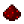# tonumberFunction tonumber Tries to convert its argument to a number. If the argument is already a number or a string convertible to a number, then tonumber returns this number; otherwise, it returns nil. An optional argument specifies the base to interpret the numeral. The base may be any integer between 2 and 36, inclusive. In bases above 10, the letter 'A' (in either upper or lower case) represents 10, 'B' represents 11, and so forth, with 'Z' representing 35. In base 10 (the default), the number can have a decimal part, as well as an optional exponent part. In other bases, only unsigned integers are accepted. Syntax tonumber(string number [, number base]) Returns None Part of ComputerCraft API noneExample Converts strings into numbers and adds them together. (It would be more intelligent to just save it as a number.) Code ```local number1 = "10" local number2 = "5" print(number1, " + ", number2, " = ", tonumber(number1) + tonumber(number2)) ``` Output 10 + 5 = 15